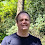### Java Intrinsics and Performance

The original question was How to count the number of 1's a number will have in binary? I included a performance comparison of using Integer.bitCount() which can be turned into an intrinic i.e. a single machine code instruction POPCNT and the Java code which does the same thing.

## Question

How do I count the number of `1`'s a number will have in binary?
So let's say I have the number `45`, which is equal to `101101` in binary and has 4 `1`'s in it. What's the most efficient way to write an algorithm to do this?

Instead of writing an algorithm to do this it's best to use the built in function. Integer.bitCount()

What makes this especially efficient is that the JVM can treat this as an intrinsic. i.e. recognise and replace the whole thing with a single machine code instruction on a platform which supports it e.g. Intel/AMD

To demonstrate how effective this optimisation is

``````public static void main(String... args) {
perfTestIntrinsic();

perfTestACopy();
}

private static void perfTestIntrinsic() {
long start = System.nanoTime();
long countBits = 0;
for (int i = 0; i < Integer.MAX_VALUE; i++)
countBits += Integer.bitCount(i);
long time = System.nanoTime() - start;
System.out.printf("Intrinsic: Each bit count took %.1f ns, countBits=%d%n", (double) time / Integer.MAX_VALUE, countBits);
}

private static void perfTestACopy() {
long start2 = System.nanoTime();
long countBits2 = 0;
for (int i = 0; i < Integer.MAX_VALUE; i++)
countBits2 += myBitCount(i);
long time2 = System.nanoTime() - start2;
System.out.printf("Copy of same code: Each bit count took %.1f ns, countBits=%d%n", (double) time2 / Integer.MAX_VALUE, countBits2);
}

// Copied from Integer.bitCount()
public static int myBitCount(int i) {
// HD, Figure 5-2
i = i - ((i >>> 1) & 0x55555555);
i = (i & 0x33333333) + ((i >>> 2) & 0x33333333);
i = (i + (i >>> 4)) & 0x0f0f0f0f;
i = i + (i >>> 8);
i = i + (i >>> 16);
return i & 0x3f;
}``````

prints

``````Intrinsic: Each bit count took 0.4 ns, countBits=33285996513
Copy of same code: Each bit count took 2.4 ns, countBits=33285996513``````

Each bit count using the intrinsic version and loop takes just 0.4 nano-second on average. Using a copy of the same code takes 6x longer (gets the same result)

1.How to find out whether any particular method intrinsic or not?

2.In the past, I have dumped the machine code generated to check that some of the Unsafe native methods are turned into intrinsic. I imagine there is a better/simpler way to do this but I don't know of any. Perhaps a look at the source code??

3.I thing I found the answer. You are right, all intrinsic are listed here: http://hg.openjdk.java.net/jdk8/awt/hotspot/file/d61761bf3050/src/share/vm/classfile/vmSymbols.hpp.

4.It's worth remembering that intrinsics behave differently on different JVMs, Android and JRockit are particularly bad AFAIK.

5.@mitsan I agree that intrinsics are very JVM specific and can change between versions.

6.+= operation is taking most of the time, rather than the any of those bitcount functions